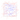# Buffon’s Needle

14 March 2009 — written by Prakash PrasadBuffon’s needle is a classic experiment in the field of Geometric Probability Theory.

A needle of length $1$ is thrown on a square floor tile with sides of length $2$. The problem is to determine the probability $p$ of the event that the needle will fall across a crack between planks.

Using the geometry, we can calculate $p=\frac{1}{\pi}$

We can physically perform this experiment, or simulate it on a computer.

Suppose we throw the needle $N=160$ times. We count the number of times $M$ that the needle falls across a crack between planks. In our computer simulation this event (red needle) happened $M=49$ times and did not happen (blue needle) $111$ times.

Hence,

$\frac{M}{N}=p=\frac{1}{\pi}$

i.e., with the values from our experiment:

$\pi=\frac{N}{M}=\frac{160}{49}=3.26531$

This approximation is an example of the general mathematical technique called the Monte Carlo Method. Monte Carlo methods are widely applied to computer simulations, especially computer games. The problem really is that while theorizing probabilities of chance is perfectly fine, when it comes to practical application, the probabilities are different over different sample space. For example, even thought the probability of tossing a coin is $0.5$ for each face, when an actual coin is picked and tossed over different number of times, the probabilities may not match the theoretical value.

Hence, computer simulations of these weighted natural outcomes are run to get a value for the probability of an occurrence which helps game designers in balancing the game.